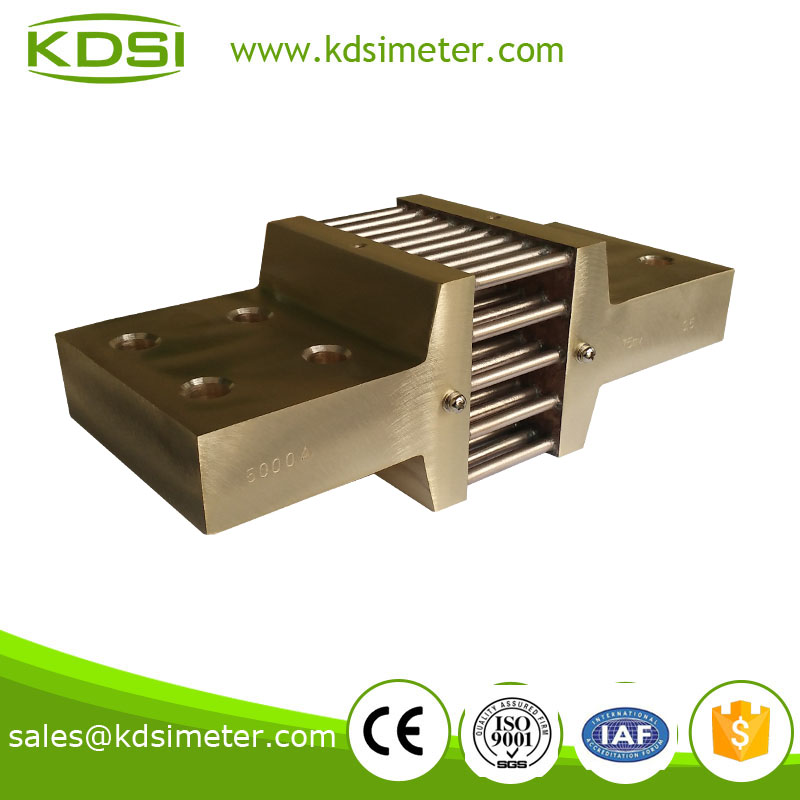Service
Hotline
+86-512-57458991
Email: sales@kdsimeter.com
You are here: » » » DC Current Divider Shunt Resistor for ammeter ampere panel meter

# DC Current Divider Shunt Resistor for ammeter ampere panel meter

Views: 75     Author: KDS INSTRUMENT(KUNSHAN)CO.,LTD     Publish Time: 2017-06-26      Origin: www.kdsimeter.comDC Current Divider Shunt Resistor for ammeter ampere panel meter

★ The use of shunt

The shunt is based on the principle of voltage on both ends of the resistor according to the current

╠ 1.     The shunt is widely used to expand the range of measuring current of ammetert, which can be used in communication system, electronic whole machine, automatic control power supply and other circuits to restrict flow, all flow sampling inspection.

2.     The shunt is used to measure dc current.

╠ 3.     The shunt is actually a resistor with a small resistance, when electric current flows, it will generate a voltage drop for dc ammeter. The dc current meter is actually a voltmeter with a full value of 75mV. Dc ammeter and shunt are match and use.

4.     The shunt is a precise resistance that can be passed through a large current.

※ Measurement of shunt

How do you measure a large dc current, such as dozens of amperes, or even larger, hundreds of amperes, without such a large range of ammeter?

Here we need a shunt.The shunt is a precise resistance that can be passed through a large current. When a current flows through the shunt, on both ends of it will appear a millivolt voltage level, then use a millivolt voltage meter to measure the voltage, then the voltage conversion into current. Completes the high current measurement.

The current meter has many different specifications, but the actual movement is the standard millivolt voltmeter. For example, a voltmeter with a full scale of 75mv. Then, measuring the current of 20A with this voltmeter, it needs to be matched with a shunt resistor that produces a voltage drop of 75mv when it flows through the 20A current, also known as the 75mv shunt.

Shunt is a great to current through the resistance of the 15A or 20A usually used a and 35A all need shunt ammeter, shunt impedance = sign full voltage meter/full current meter. Such as 20A shunt resistance of ammeter = 75 * 10 mv - 3/20A = 0.00375 Ω, constant impedance according to ohm's law after U = IR, current is proportional to the voltage and current for the linear voltage is linear, So you can display current using a voltmeter with a full length of 75mv. Therefore, the current meter used is actually a voltmeter.

※ The methods for selection of shunt

1. 1)    Select the rated voltage drop specification of the shunt (commonly used in 75mV or 45mV) according to the number of mV selected by the current meter (or current voltmeter) dial. If there is no such value in the current meter, the voltage limit of the calculated meter is used, then the rated voltage of the shunt is selected.

The meter voltage limit (mV) = current meter when the full scale of current (A) internal resistance of ammeter (Ω) / 1000

1. 2)    The rated current specification of the shunt is selected for the current range of the current range.

2. 3)    It will be selected two shunt current terminal connected to the power and load, respectively, potential termination ammeter, should pay attention to the terminal polarity to the ammeter, the range of the ammeter is expanded to shunt calibration on the current value.